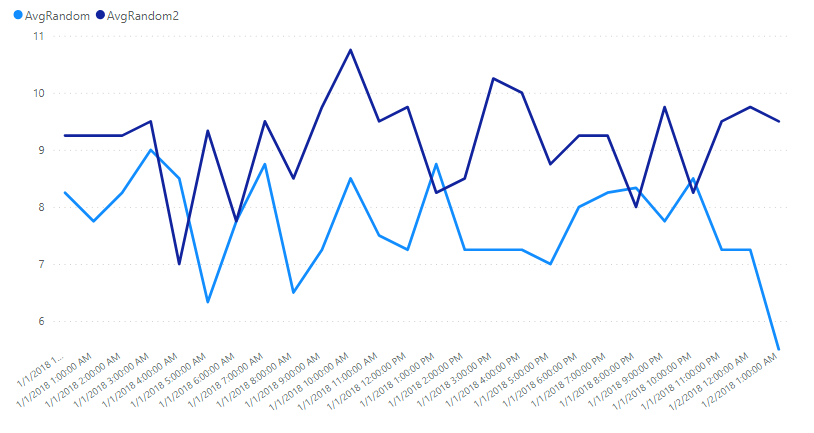# Time Tables in Power BI

In a previous video, the importance and benefits of splitting DateTime columns into separate Date and Time columns was discussed. For example, a table with DateTime columns of StartDateTime and StopDateTime should be transformed in the query editor to the below.

You can then add a date table and a time table to your model. In this example, the active relationship is to StartTime and inactive is to StopTime.

There are many examples out there for date tables, but not as many for time tables (this makes sense, as you should eliminate the time component whenever only day-level analysis is needed). A previous article provides date tables (in either DAX or M) that do not require any Sort-By Columns.

Below is the DAX or M code to generate a typical time table, with several useful dimensions (both create the same table). The tables generated by the code below are at the minute-level granularity (one row for every minute in a day). Examples to adjust the granularity by changing the first line are shown below each.

M Time Table

``````let
Source = List.Times(#time(0, 0, 0), 24 * 60, #duration(0, 0, 1, 0)),
#"Converted to Table" = Table.FromList(Source, Splitter.SplitByNothing(), null, null, ExtraValues.Error),
#"Renamed Columns" = Table.RenameColumns(#"Converted to Table", {{"Column1", "Time"}}),
#"Changed Type" = Table.TransformColumnTypes(#"Renamed Columns", {{"Time", type time}}),
#"Inserted Hour" = Table.AddColumn(#"Changed Type", "Hour", each Time.Hour([Time]), Int64.Type),
#"Inserted Start of Hour" = Table.AddColumn(#"Inserted Hour", "Start of Hour", each Time.StartOfHour([Time]), type time),
Added_HalfHrs = Table.AddColumn(#"Inserted Start of Hour", "HalfHr", each #time(Time.Hour([Time]), 30 * Number.RoundDown(Time.Minute([Time]) / 30), 0), type time),
"TimeOfDay",
each
if [Hour] >= 6 and [Hour] <= 11 then
Text.Repeat(Character.FromNumber(8203), 3) & "Morning"
else if [Hour] >= 12 and [Hour] <= 17 then
Text.Repeat(Character.FromNumber(8203), 2) & "Afternoon"
else if [Hour] >= 18 and [Hour] <= 23 then
Text.Repeat(Character.FromNumber(8203), 1) & "Evening"
else
Text.Repeat(Character.FromNumber(8203), 4) & "Night",
type text
),
in

Example Changes

Source = List.Times(#time(0, 0, 0), 24 * 60 * 60, #duration(0, 0, 0, 1)) // 1 second granularity

Source = List.Times(#time(0, 0, 0), 24 * 60 / 5, #duration(0, 0, 5, 0)) // 5 minute granularity

DAX Time Table

``````Time_DAX =
VAR basetime =
SELECTCOLUMNS (
GENERATESERIES ( TIME ( 0, 0, 0 ), TIME ( 23, 59, 0 ), TIME ( 0, 1, 0 ) ),
"Time", [Value]
)
VAR output =
basetime,
"Hour", HOUR ( [Time] ),
"AM/PM", IF ( HOUR ( [Time] ) >= 12, "PM", "AM" ),
"TimeOfDay",
SWITCH (
TRUE (),
HOUR ( [Time] ) >= 18, REPT ( UNICHAR ( 8203 ), 1 ) & "Evening",
HOUR ( [Time] ) >= 12, REPT ( UNICHAR ( 8203 ), 2 ) & "Afternoon",
HOUR ( [Time] ) >= 6, REPT ( UNICHAR ( 8203 ), 3 ) & "Morning",
REPT ( UNICHAR ( 8203 ), 4 ) & "Night"
),
"Shift",
SWITCH (
TRUE (),
HOUR ( [Time] ) >= 16, "2nd",
HOUR ( [Time] ) >= 8, "1st",
"3rd"
),
"StartOfHour", TIME ( HOUR ( [Time] ), 0, 0 ),
"HalfHours",
TIME ( HOUR ( [Time] ), 30 * ROUNDDOWN ( MINUTE ( [Time] ) / 30, 0 ), 0 ),
"QtrHours",
TIME ( HOUR ( [Time] ), 15 * ROUNDDOWN ( MINUTE ( [Time] ) / 15, 0 ), 0 )
)
RETURN
output
``````

Example Changes

GENERATESERIES ( TIME ( 0, 0, 0 ), TIME ( 23, 59, 59 ), TIME ( 0, 0, 1 ) ) // 1 second granularity

GENERATESERIES ( TIME ( 0, 0, 0 ), TIME ( 23, 55, 0 ), TIME ( 0, 5, 0 ) ) // 5 minute granularity

Note: The data type of the Time, StartOfHour, HalfHours, and QtrHours needs to be changed to Time when you first create the DAX table.

Visualizing Time Series Data With Split Date and Time Tables

If your visual just covers one day, you can simply use the Time column from the Time table. However, since a DateTime column no longer exists, you can use both the Date (from the Date table) and the Time (from the Time table) in a hierarchy as demonstrated below.Add a column from the Date and Time tables and then hit the double-down arrow on visual ("Expand all down one level in the hierarchy") to get the date and time granularity in a line chart (for example)The StartOfHour column from the Time table was used so that more than one day would be visible on X axis.

Alternatively, you can create a measure that combines the date and time values, and then use a visual that allows measure for axes/legends like Charticulator or Deneb.

I hope you find this article useful. It doesn't matter which one you use (DAX or M). I tend to use the M one, but use the one you feel more comfortable adapting to fit your needs. It is important to use as high a granularity as possible for your data (Days > Hours > Minutes > Seconds). The more rows you have to iterate over for your analyses, the worse performance will be.

### You May Also Like#### About the Author: Pat Mahoney

Pat Mahoney is a chemist in Indiana who enjoys teaching others to use Power BI and other tools, and providing pro bono data science help/instruction to nonprofits, schools, etc.